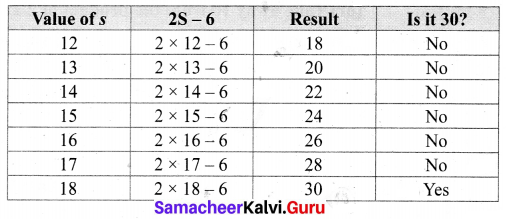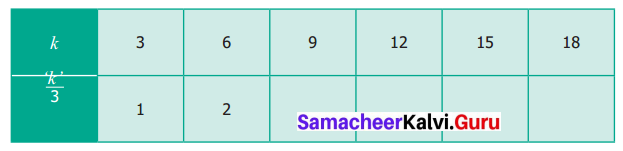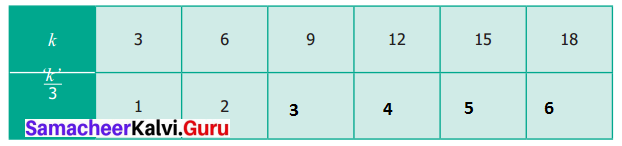## Tamilnadu Samacheer Kalvi 6th Maths Solutions Term 1 Chapter 2 Introduction to Algebra Ex 2.2Question 1.
Find in the blanks.
(i) The algebraic statement of ‘f’ decreased by 5 is _______
(ii) The algebraic statement of ‘s’ divided by 5 is _______
(iii) The verbal statement of ‘2m – 10’ is _______
(iv) If A’s age is ‘n’ years now, 7 years ago A’s age was ______
(v) If ‘p – 5’ gives 12 then ‘p’ is ______
Solution:
(i) f – 5
(ii) $$\frac { s }{ 5 }$$
(iii) 10 less than 2 times m (or) Take away 10 from the product of 2 and m
(iv) n – 7
(v) 17
Hint: n – 5 = 12⇒ n = 12 + 5 = 17

Question 2.
Say True or False.
(i) 10 more to three times ‘c’ is ‘3c + 13’.
(ii) If the cost of 10 rice bags is Rs ‘t’, then the cost of 1 rice bag is Rs $$\frac{t}{10}$$
(iii) The statements ‘x’ divided by 3 and 3 divided by ‘x’ are the same.
(iv) The product of ‘q’ and 20 is ‘20q’.
(v) 7 less to 7 times ‘y’ is ‘7 – 7y’.
Solution:
(i) False
(ii) True
(iii) False
(iv) True
(v) False

Question 3.
Express the following verbal statement to algebraic statement.
(i) ‘t’ is added to 100
(ii) 4 times ‘q’
(iii) 8 is reduced by ‘y’
(iv) 56 added to 2 times ‘x’
(v) 4 less to 9 times of ‘y’
Solution:
(i) t + 100
(ii) 4q
(iii) 8 – y
(iv) 2x + 56
(v) 9y – 4Question 4.
Express the following algebraic statement to verbal statement.
(i) x ÷ 3
(ii) 5n – 12
(iii) 11 + 10x
(iv) 70s
Solution:
(i) x divided by 3
(ii) 12 less to 5 times n
(iii) 11 added to 10 times x
(iv) 70 times s

Question 5.
The teacher asked two students to write the algebraic statement for the verbal statement “8 more than a number” on the board. Vetri wrote ‘8 + x’ but Maran wrote ‘8x’. Who gave the correct answer?
Solution:
Let the number be x; 8 more than the number = 8 + x.
Vetri gave the correct answer as 8 + x.

Question 6.
(i) If ‘n’ takes the value 3 then find the value of ‘n + 10’
(ii) If ‘g’ is equal to 300. What is the value of ‘g – 1’ and ‘g + 1’?
(iii) What is the value of s; if ‘2s – 6’ gives 30?
Solution:
(i) Given n = 3
then n + 10 = 3 + 10 = 13
n + 10 = 13

(ii) Given g = 300
g – 1 = 300 – 1 = 299
g + 1 = 300 + 1 = 301
g – 1 = 299; g + 1 = 301

(iii) Given 2s – 6 = 30
From the table:
2 × 18 – 6 = 30
s = 18Question 7.
Complete the table and find the value of ‘k’ for which $$\frac { k }{ 3 }$$ gives 5.Solution:
Given: k ÷ 3 = 5 From the above table we find that
15 ÷ 3 = 5
k = 5Objective Type Questions

Question 8.
The value of ‘y’ in y + 7 = 13 is
(a) y = 5
(b) y = 6
(c) y = 7
(d) y = 8
Solution:
(b) y = 6

Question 9.
6 less to ‘n’ gives 8 is represented as
(a) n – 6 = 8
(b) 6 – n = 8
(c) 8 – n = 6
(d) n – 8 = 6
Solution:
(a) n – 6 = 8

Question 10.
The value of ‘c’ for which $$\frac{3c}{4}$$ gives 18 is
(a) c = 15
(b) c = 21
(c) c = 24
(d) c = 27
Solution:
(c) c = 24